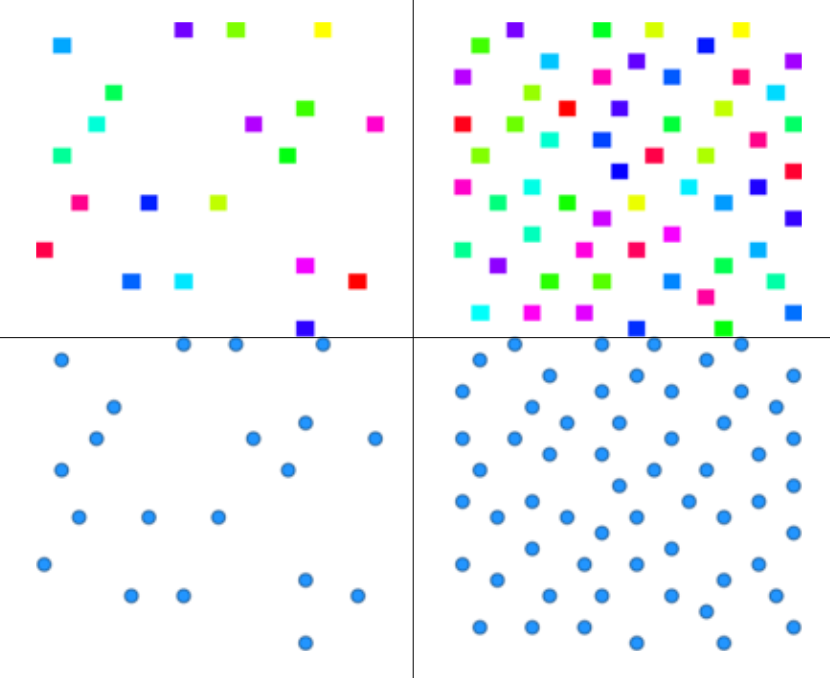Note: This document is for an older version of GRASS GIS that will be discontinued soon. You should upgrade, and read the current manual page.

## NAME

r.random.cells - Generates random cell values with spatial dependence.

## KEYWORDS

raster, sampling, random, autocorrelation

## SYNOPSIS

r.random.cells
r.random.cells --help
r.random.cells output=name distance=float [ncells=integer] [seed=integer] [--overwrite] [--help] [--verbose] [--quiet] [--ui]

### Flags:

--overwrite
Allow output files to overwrite existing files
--help
Print usage summary
--verbose
Verbose module output
--quiet
Quiet module output
--ui
Force launching GUI dialog

### Parameters:

output=name [required]
Name for output raster map
distance=float [required]
Maximum distance of spatial correlation (value >= 0.0)
ncells=integer
Maximum number of cells to be created
Options: 1-
seed=integer
Random seed, default [random]

## DESCRIPTION

r.random.cells generates a random sets of raster cells that are at least distance apart. The cells are numbered from 1 to the numbers of cells generated, all other cells are NULL (no data). Random cells will not be generated in areas masked off.

### Detailed parameter description

output
Random cells. Each random cell has a unique non-zero cell value ranging from 1 to the number of cells generated. The heuristic for this algorithm is to randomly pick cells until there are no cells outside of the chosen cell's buffer of radius distance.
distance
Determines the minimum distance the centers of the random cells will be apart.
seed
Specifies the random seed that r.random.cells will use to generate the cells. If the random seed is not given, r.random.cells will get a seed from the process ID number.

## NOTES

The original purpose for this program was to generate independent random samples of cells in a study area. The distance value is the amount of spatial autocorrelation for the map being studied.

## EXAMPLES

### Random cells in given distances

North Carolina sample dataset example:
```g.region n=228500 s=215000 w=630000 e=645000 res=100 -p
r.random.cells output=random_500m distance=500
```

### Limited number of random points

Here is another example where we will create given number of vector points with the given minimal distances. Let's star with setting the region (we use large cells here):
```g.region raster=elevation
g.region rows=20 cols=20 -p
```
Then we generate random cells and we limit their count to 20:
```r.random.cells output=random_cells distance=1500 ncells=20 seed=200
```
Finally, we convert the raster cells to points using r.to.vect module:
```r.to.vect input=random_cells output=random_points type=point
```
An example of the result is at the Figure below on the left in comparison with the result without the cell limit on the right.

Additionally, we can use v.perturb module to add random spatial deviation to their position so that they are not perfectly aligned with the grid. We cannot perturb the points too much, otherwise we might seriously break the minimal distance we set earlier.

```v.perturb input=random_points output=random_points_moved parameters=50 seed=200
```
In the above examples, we were using fixed seed. This is advantageous when we want to generate (pseudo) random data, but we want to get reproducible results at the same time.Figure: Generated cells with limited number of cells (upper left), derived vector points (lower left), cells without a count limit (upper right) and corresponding vector points (lower right)

## REFERENCES

Random Field Software for GRASS GIS by Chuck Ehlschlaeger

As part of my dissertation, I put together several programs that help GRASS (4.1 and beyond) develop uncertainty models of spatial data. I hope you find it useful and dependable. The following papers might clarify their use:

r.random.surface, r.random, v.random, r.to.vect, v.perturb

## AUTHOR

Charles Ehlschlaeger; National Center for Geographic Information and Analysis, University of California, Santa Barbara.

## SOURCE CODE

Available at: r.random.cells source code (history)

Latest change: Tuesday Apr 20 08:05:18 2021 in commit: 0f706adc364538f73ea891c0c426ef763825721b

Note: This document is for an older version of GRASS GIS that will be discontinued soon. You should upgrade, and read the current manual page.

© 2003-2023 GRASS Development Team, GRASS GIS 7.8.8dev Reference Manual